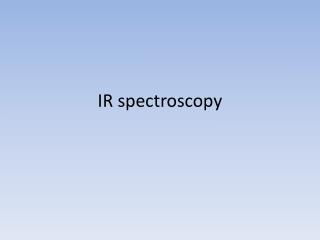Download PresentationIR spectroscopy

# IR spectroscopy - PowerPoint PPT PresentationDownload Presentation## IR spectroscopy

- - - - - - - - - - - - - - - - - - - - - - - - - - - E N D - - - - - - - - - - - - - - - - - - - - - - - - - - -
##### Presentation Transcript

1. IR spectroscopy

2. Electromagnetic Radiation • Radiation is absorbed & emitted in photons. The defining characteristic of a photon is that the energy is quantized, it can not be split into smaller pieces. • Each photon’s energy is defined by its frequency, (ν) or wave length, (λ) or wave number (wn) • Ephoton = hν = hc/λ = hc(wn) • Two constants appear in these formulas: h = 6.63 x x 10-34 J s c = speed of light = 3.00 x 108 ms-1 (3.0 x 1010cms-1) • Wave number (wn) = 1/λ

3. IR Radiation • IR absorbed by organic molecules • 600 - 4000 cm- -1 1 • Photon energy = hc(wn) = 1.2 - 8.0 x 10-20 J • Molar photon energy = 7.4 -49 kJ/mol = 1.8 -12 kcal/mol • IR photon energy <<< covalent bond energy. • Absorbing IR radiation should not trigger substantial chemical changes. But IR radiation contains more energy than random thermal motion at room temperature (~ 0.6 kcal/mol).

4. How is a spectrum created?

5. IR Spectrum • Plot IR energy vs. %transmittance (%T) • Energy scale in wave numbers, wn (cm -1) • %T scale • Compares intensity of IR striking sample (Iin) with intensity of IR leaving sample (Iout) • 100%T means no light absorbed by sample • 0% T means all light absorbed by sample

6. Energy Trends • Energy follows vibration frequency of atoms • Light atoms vibrate more rapidly • CH, NH, OH vibrations > 2800 cm -1 • Multiple bonds vibrate more rapidly • Triple bonds • C≡C (2100 - 2200) • C≡N (2240 - 2280) • Double bonds • C=O (1680 -1750) • C=C (1620 -1680) • Single bonds Single bonds • C–O (1025 - 1200) • Stretching > Bending > Wagging/Twisting

7. Interpreting a spectrum

8. Fingerprint Region